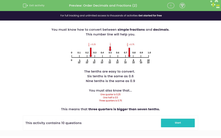# Place Decimals and Fractions in Order of Size

In this worksheet, students will use a number line to place simple fractions and decimals in order of size.Key stage:  KS 2

Curriculum topic:   Number: Fractions and Decimals

Curriculum subtopic:   Recognise Decimal Equivalents

Difficulty level:#### Worksheet Overview

We need to know how to convert between simple fractions and decimals.The tenths are easy to convert.

Six tenths is the same as 0.6

Nine tenths is the same as 0.9

We also need to know that:

One quarter is 0.25

One half is 0.5

Three quarters is 0.75

This means that three quarters is bigger than seven tenths.In this activity, we will be placing  fractions and decimals in order of size after converting them all into decimals.